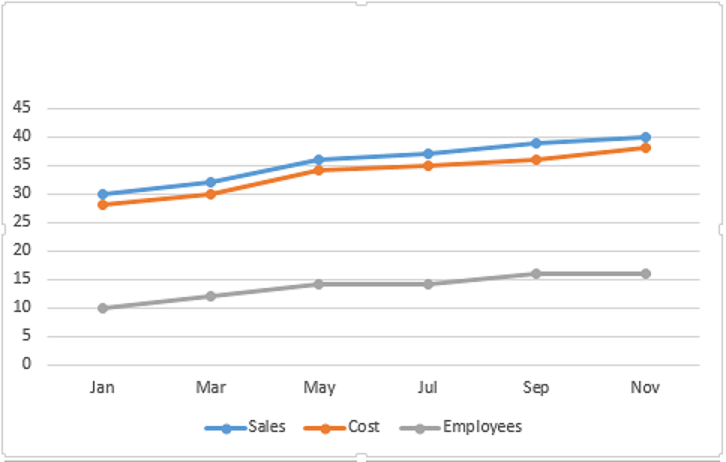### CAT 1996 Question Paper Question 66

Instructions

Answer the questions based on the following graph.Employess in thousand Sales - cost = profit
(all values are integers)

Question 66

# Which month records the highest profit percentage?

Solution

As it is given that profit = sales - cost

So profit for september = $$\frac{3}{36} \times 100$$ = 8% (Nearly)

profit for march = $$\frac{3}{30} \times 100$$ = 10% (nearly)

profit for july = $$\frac{2}{35} \times 100$$ < 10%

profit for may = $$\frac{2}{36} \times 100$$ <10%

So highest percentage profit is for march month.

• All Quant CAT Formulas and shortcuts PDF
• 30+ CAT previous papers with solutions PDF